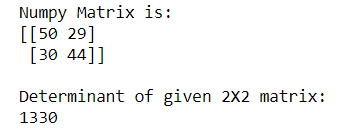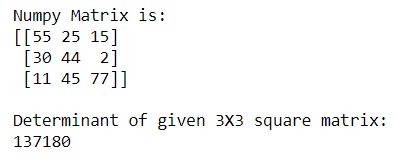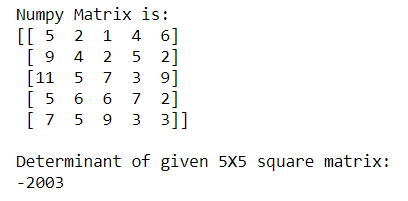# How to Calculate the determinant of a matrix using NumPy?

• Last Updated : 05 Sep, 2020

A special number that can be calculated from a square matrix is known as the Determinant of a square matrix. The Numpy provides us the feature to calculate the determinant of a square matrix using numpy.linalg.det() function.

Syntax:

Attention geek! Strengthen your foundations with the Python Programming Foundation Course and learn the basics.

To begin with, your interview preparations Enhance your Data Structures concepts with the Python DS Course. And to begin with your Machine Learning Journey, join the Machine Learning - Basic Level Course

```numpy.linalg.det(array)
```

Example 1: Calculating Determinant of a 2X2 Numpy matrix using numpy.linalg.det() function

## Python3

 `# importing Numpy package``import` `numpy as np`` ` `# creating a 2X2 Numpy matrix``n_array ``=` `np.array([[``50``, ``29``], [``30``, ``44``]])`` ` `# Displaying the Matrix``print``(``"Numpy Matrix is:"``)``print``(n_array)`` ` `# calculating the determinant of matrix``det ``=` `np.linalg.det(n_array)`` ` `print``(``"\nDeterminant of given 2X2 matrix:"``)``print``(``int``(det))`

Output:In the above example, we calculate the Determinant of the 2X2 square matrix.

Example 2: Calculating Determinant of a 3X3 Numpy matrix using numpy.linalg.det() function

## Python3

 `# importing Numpy package``import` `numpy as np`` ` `# creating a 3X3 Numpy matrix``n_array ``=` `np.array([[``55``, ``25``, ``15``],``                    ``[``30``, ``44``, ``2``],``                    ``[``11``, ``45``, ``77``]])`` ` `# Displaying the Matrix``print``(``"Numpy Matrix is:"``)``print``(n_array)`` ` `# calculating the determinant of matrix``det ``=` `np.linalg.det(n_array)`` ` `print``(``"\nDeterminant of given 3X3 square matrix:"``)``print``(``int``(det))`

Output:In the above example, we calculate the Determinant of the 3X3 square matrix.

Example 3: Calculating Determinant of a 5X5 Numpy matrix using numpy.linalg.det() function

## Python3

 `# importing Numpy package``import` `numpy as np`` ` `# creating a 5X5 Numpy matrix``n_array ``=` `np.array([[``5``, ``2``, ``1``, ``4``, ``6``],``                    ``[``9``, ``4``, ``2``, ``5``, ``2``],``                    ``[``11``, ``5``, ``7``, ``3``, ``9``],``                    ``[``5``, ``6``, ``6``, ``7``, ``2``],``                    ``[``7``, ``5``, ``9``, ``3``, ``3``]])`` ` `# Displaying the Matrix``print``(``"Numpy Matrix is:"``)``print``(n_array)`` ` `# calculating the determinant of matrix``det ``=` `np.linalg.det(n_array)`` ` `print``(``"\nDeterminant of given 5X5 square matrix:"``)``print``(``int``(det))`

Output:In the above example, we calculate the Determinant of the 5X5 square matrix.

My Personal Notes arrow_drop_up